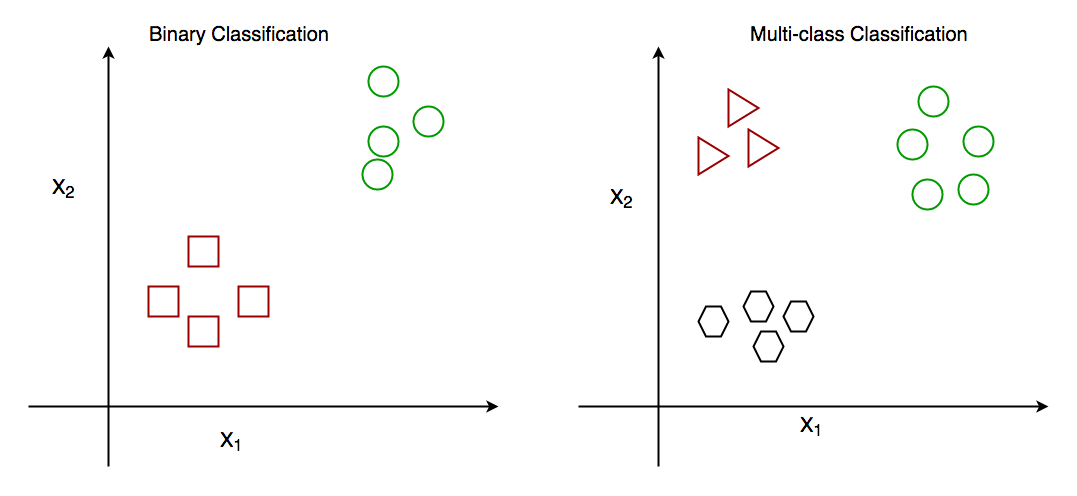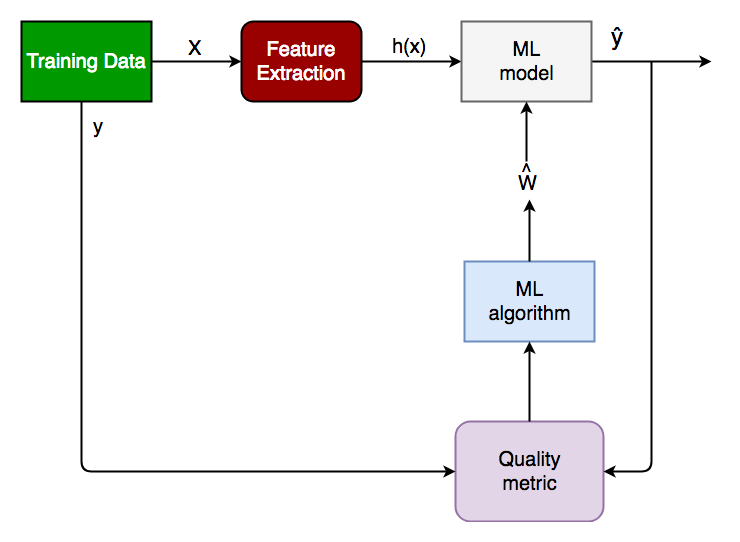GFG App
Open AppBrowser
Continue

# Getting started with Classification

As the name suggests, Classification is the task of “classifying things” into sub-categories. But, by a machine! If that doesn’t sound like much, imagine your computer being able to differentiate between you and a stranger. Between a potato and a tomato. Between an A grade and an F. Now, it sounds interesting now. Classification is part of supervised machine learning in which we put labeled data for training. In Machine Learning and Statistics, Classification is the problem of identifying to which of a set of categories (subpopulations), a new observation belongs, on the basis of a training set of data containing observations and whose categories membership is known.

## What is Classification?

Classification is a process of categorizing data or objects into predefined classes or categories based on their features or attributes. In machine learning, classification is a type of supervised learning technique where an algorithm is trained on a labeled dataset to predict the class or category of new, unseen data.

The main objective of classification is to build a model that can accurately assign a label or category to a new observation based on its features. For example, a classification model might be trained on a dataset of images labeled as either dogs or cats and then used to predict the class of new, unseen images of dogs or cats based on their features such as color, texture, and shape.

### Types of Classification

Classification is of two types:

1. Binary Classification: In binary classification, the goal is to classify the input into one of two classes or categories. Example – On the basis of the given health conditions of a person, we have to determine whether the person has a certain disease or not.
2. Multiclass Classification: In multi-class classification, the goal is to classify the input into one of several classes or categories. For Example – On the basis of data about different species of flowers, we have to determine which specie our observation belongs to.Binary vs Multi class classification

### Types of classification algorithms

There are various types of classifiers. Some of them are :

• Linear Classifiers: Linear models create a linear decision boundary between classes. They are simple and computationally efficient. Some of the linear classification models are as follows:
• Non-linear Classifiers: Non-linear models create a non-linear decision boundary between classes. They can capture more complex relationships between the input features and the target variable. Some of the non-linear classification models are as follows:

### Type of Learners in Classifications Algorithm

In machine learning, classification learners can also be classified as either “lazy” or “eager” learners.

• Lazy Learners: Lazy Learners are also known as instance-based learners, lazy learners do not learn a model during the training phase. Instead, they simply store the training data and use it to classify new instances at prediction time. It is very fast at prediction time because it does not require computations during the predictions. it is less effective in high-dimensional spaces or when the number of training instances is large. Examples of lazy learners include k-nearest neighbors and case-based reasoning.
• Eager Learners: Eager Learners are also known as model-based learners, eager learners learn a model from the training data during the training phase and use this model to classify new instances at prediction time. It is more effective in high-dimensional spaces having large training datasets. Examples of eager learners include decision trees, random forests, and support vector machines.

### Classification model Evaluations

Evaluating a classification model is an important step in machine learning, as it helps to assess the performance and generalization ability of the model on new, unseen data. There are several metrics and techniques that can be used to evaluate a classification model, depending on the specific problem and requirements. Here are some commonly used evaluation metrics:

• Classification Accuracy: The proportion of correctly classified instances over the total number of instances in the test set. It is a simple and intuitive metric but can be misleading in imbalanced datasets where the majority class dominates the accuracy score.
• Confusion matrix: A table that shows the number of true positives, true negatives, false positives, and false negatives for each class, which can be used to calculate various evaluation metrics.
• Precision and Recall: Precision measures the proportion of true positives over the total number of predicted positives, while recall measures the proportion of true positives over the total number of actual positives. These metrics are useful in scenarios where one class is more important than the other, or when there is a trade-off between false positives and false negatives.
• F1-Score: The harmonic mean of precision and recall, calculated as 2 x (precision x recall) / (precision + recall). It is a useful metric for imbalanced datasets where both precision and recall are important.
• ROC curve and AUC: The Receiver Operating Characteristic (ROC) curve is a plot of the true positive rate (recall) against the false positive rate (1-specificity) for different threshold values of the classifier’s decision function. The Area Under the Curve (AUC) measures the overall performance of the classifier, with values ranging from 0.5 (random guessing) to 1 (perfect classification).
• Cross-validation: A technique that divides the data into multiple folds and trains the model on each fold while testing on the others, to obtain a more robust estimate of the model’s performance.

It is important to choose the appropriate evaluation metric(s) based on the specific problem and requirements, and to avoid overfitting by evaluating the model on independent test data.

### How does classification work?

The basic idea behind classification is to train a model on a labeled dataset, where the input data is associated with their corresponding output labels, to learn the patterns and relationships between the input data and output labels. Once the model is trained, it can be used to predict the output labels for new unseen data.

#### The classification process typically involves the following steps:

1. Understanding the problem: Before getting started with classification, it is important to understand the problem you are trying to solve. What are the class labels you are trying to predict? What is the relationship between the input data and the class labels?
• Suppose we have to predict whether a patient has a certain disease or not, on the basis of 7 independent variables, called features. This means, there can be only two possible outcomes:
• The patient has the disease, which means “True”.
• The patient has no disease. which means “False”.
• This is a binary classification problem.
2. Data preparation: Once you have a good understanding of the problem, the next step is to prepare your data. This includes collecting and preprocessing the data and splitting it into training, validation, and test sets. In this step, the data is cleaned, preprocessed, and transformed into a format that can be used by the classification algorithm.
• X: It is the independent feature, in the form of an N*M matrix. N is the no. of observations and M is the number of features.
• y: An N vector corresponding to predicted classes for each of the N observations.
3. Feature Extraction: The relevant features or attributes are extracted from the data that can be used to differentiate between the different classes.
• Suppose our input X has 7 independent features, having only 5 features influencing the label or target values remaining 2 are negligibly or not correlated, then we will use only these 5 features only for the model training.
4. Model Selection: There are many different models that can be used for classification, including logistic regression, decision trees, support vector machines (SVM), or neural networks. It is important to select a model that is appropriate for your problem, taking into account the size and complexity of your data, and the computational resources you have available.
5. Model Training: Once you have selected a model, the next step is to train it on your training data. This involves adjusting the parameters of the model to minimize the error between the predicted class labels and the actual class labels for the training data.
6. Model Evaluation: Evaluating the model: After training the model, it is important to evaluate its performance on a validation set. This will give you a good idea of how well the model is likely to perform on new, unseen data.
• Log Loss or Cross-Entropy Loss, Confusion Matrix,  Precision, Recall, and AUC-ROC curve are the quality metrics used for measuring the performance of the model.
7. Fine-tuning the model:  If the model’s performance is not satisfactory, you can fine-tune it by adjusting the parameters, or trying a different model.
8. Deploying the model: Finally, once we are satisfied with the performance of the model, we can deploy it to make predictions on new data.  it can be used for real world problem.Classification Lifecycle

### Applications of Classification Algorithm

Classification algorithms are widely used in many real-world applications across various domains, including:

• Email spam filtering
• Credit risk assessment
• Medical diagnosis
• Image classification
• Sentiment analysis.
• Fraud detection
• Quality control
• Recommendation systems

### Implementation:

Let’s get a hands-on experience with how Classification works. We are going to study various Classifiers and see a rather simple analytical comparison of their performance on a well-known, standard data set, the Iris data set.

Requirements for running the given script:

Example

## Python

 `# Importing the required libraries` `import` `numpy as np` `import` `pandas as pd` `from` `sklearn.model_selection ``import` `train_test_split` `from` `sklearn.metrics ``import` `accuracy_score` `from` `sklearn ``import` `datasets` `from` `sklearn ``import` `svm` `from` `sklearn.tree ``import` `DecisionTreeClassifier` `from` `sklearn.naive_bayes ``import` `GaussianNB`   `# import the iris dataset` `iris ``=` `datasets.load_iris()` `X ``=` `iris.data` `y ``=` `iris.target`   `# splitting X and y into training and testing sets` `X_train, X_test, y_train, y_test ``=` `train_test_split(` `    ``X, y, test_size``=``0.3``, random_state``=``1``)`   `# GAUSSIAN NAIVE BAYES` `gnb ``=` `GaussianNB()` `# train the model` `gnb.fit(X_train, y_train)` `# make predictions` `gnb_pred ``=` `gnb.predict(X_test)` `# print the accuracy` `print``(``"Accuracy of Gaussian Naive Bayes: "``,` `      ``accuracy_score(y_test, gnb_pred))`   `# DECISION TREE CLASSIFIER` `dt ``=` `DecisionTreeClassifier(random_state``=``0``)` `# train the model` `dt.fit(X_train, y_train)` `# make predictions` `dt_pred ``=` `dt.predict(X_test)` `# print the accuracy` `print``(``"Accuracy of Decision Tree Classifier: "``,` `      ``accuracy_score(y_test, dt_pred))`   `# SUPPORT VECTOR MACHINE` `svm_clf ``=` `svm.SVC(kernel``=``'linear'``)  ``# Linear Kernel` `# train the model` `svm_clf.fit(X_train, y_train)` `# make predictions` `svm_clf_pred ``=` `svm_clf.predict(X_test)` `# print the accuracy` `print``(``"Accuracy of Support Vector Machine: "``,` `      ``accuracy_score(y_test, svm_clf_pred))`

Output:

```Accuracy of Gaussian Naive Bayes:  0.9333333333333333
Accuracy of Decision Tree Classifier:  0.9555555555555556
Accuracy of Support Vector Machine:  1.0```

### Conclusion:

Classification is a very vast field of study. Even though it comprises a small part of Machine Learning as a whole, it is one of the most important ones.
That’s all for now. In the next article, we will see how Classification works in practice and get our hands dirty with Python Code.

My Personal Notes arrow_drop_up